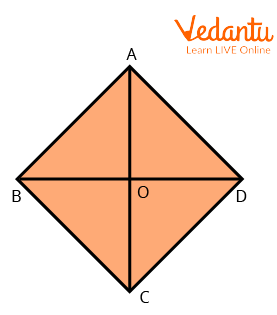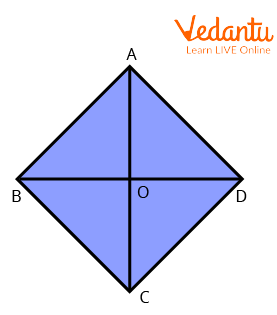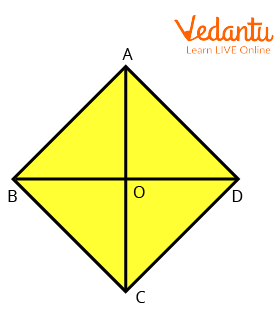Courses
Courses for Kids
Free study material
Free LIVE classes
More

# What is the Perpendicular Line Theorem?LIVE
Join Vedantu’s FREE Mastercalss

## Perpendicular Line Theorem: Brief Introduction

The perpendicular Line Theorem is a fundamental tool of Euclidean Geometry and is used to derive the various properties of perpendicular lines. In this article, we will discuss the proof of the theorem and its importance in geometry. Perpendicular lines are the lines which intersect each other at 90 degrees. So, in this article, we will discuss the properties of such lines and the examples related to the perpendicular line theorem. Here, the theorem will be proved with the help of figures and diagrams.

## Statement of Perpendicular Line Theorem

According to the Perpendicular Line Theorem, if two straight lines are intersecting each other at a point and forming a linear pair of equal angles at that point, then the lines are perpendicular to each other.

## Proof of Perpendicular Line TheoremPerpendicular Line Theorem

Let AB and CD be the lines intersecting at point O.

According to the statement of the Perpendicular Lines Theorem,

AB and CD make a pair of equal angles at O.

i.e.,

\$\angle 1=\angle 2\$

Also, we know from linear pair angles property,

\$\angle 1+\angle 2=180^{\circ}\$

Putting \$\angle 1=\angle 2\$, we get

\$\therefore \angle 1=\angle 2=\dfrac{180}{2}=90^{\circ}\$

Or we can say that lines AB and CD are perpendicular to each other as the angle between them is 90 degrees.

Hence Proved.

## Limitations of Perpendicular Line Theorem

• The Perpendicular Line theorem doesn’t state anything if the pair of angles are not equal.

• It does not apply to any other types of line such as parallel lines.

## Applications of Perpendicular Line Theorem

• The Perpendicular Line Theorem has a wide range of applications in construction as the walls of buildings are always perpendicular to each other.

• The Perpendicular Line Theorem forms one of the fundamental tools of geometry and helps in solving geometry problems.

## Solved Examples

1. In the quadrilateral \$A B C D, A C\$ and \$B D\$ make an equal pair of angles and \$\angle O A D=30^{\circ}\$. Find \$\angle A D O\$.Ans: Using the perpendicular line theorem,

\$\angle A O D=90^{\circ}\$

And we know

\$\Rightarrow \angle O A D+\angle A O D+\angle A D O=180^{\circ}\$

Putting the values we get,

\$\Rightarrow 30^{\circ}+90^{\circ}+\angle A D O=180^{\circ} \$

\$\Rightarrow \angle A D O=180^{\circ}-120^{\circ}\$

\$\Rightarrow \angle A D O=60^{\circ}\$

2. In the quadrilateral \$A B C D, A C\$ and \$B D\$ make an equal pair of angles and \$\angle O A D=60^{\circ}\$. Find \$\angle A D O\$.Ans: Using the perpendicular line theorem,

\$\angle A O D=90^{\circ}\$

And we know

\$\Rightarrow \angle O A D+\angle A O D+\angle A D O=180^{\circ}\$

Putting the values we get,

\$\Rightarrow \angle A D O=+180^{\circ}-150^{\circ}\$

\$\angle A D O=30^{\circ}\$

3. In the quadrilateral \$A B C D, A C\$ and \$B D\$ make an equal pair of angles and \$\angle O C D=45^{\circ}\$. Find \$\angle C D O\$.Ans: Using the perpendicular line theorem,

\$\angle C O D=90^{\circ}\$

And we know

\$\Rightarrow \angle O C D+\angle C O D+\angle C D O=180^{\circ}\$

Putting the values we get,

\$\Rightarrow 45^{\circ}+90^{\circ}+\angle C D O=180^{\circ} \$

\$\Rightarrow \angle C D O=180^{\circ}-135^{\circ} \$

\$\Rightarrow \angle C D O=45^{\circ}\$

## Important Points to Remember

• Lines making equal pairs of angles at intersections are perpendicular to each other.

• If the angles formed between the lines are 90 degrees, then lines are said to be perpendicular lines.

## Important Formulas to Remember

• Two lines \$AB\$ and \$CD\$ are perpendicular to each other if the angle between them is \$90\$ degrees.

## Conclusion

In the article, we have discussed the detailed proof of the Perpendicular Line Theorem and its applications. We have observed from the above discussions that the perpendicular line theorem is of great use in geometry and reduces our work in solving problems with its application. In all, we can say that it is a theorem of great significance for us.

Last updated date: 19th Sep 2023
Total views: 100.2k
Views today: 2.00k

## FAQs on What is the Perpendicular Line Theorem?

1. What are the properties of perpendicular Lines?

Two distinct lines are said to be perpendicular if they intersect each other at 90 degrees. We can simply recognize perpendicular lines by simply looking at them. If the figure is an ‘L’ shaped figure, then the lines are perpendicular to each other. The two most important properties of Perpendicular Lines are as follows:

• If two lines are perpendicular, then they will always intersect each other.

• The angle of intersection between the two perpendicular lines is always equal to 90 degrees.

2. What are the different methods to draw perpendicular lines?

Perpendicular lines can be drawn in two different ways.

Using a Compass:

2. Place the compass at a fixed point on a line on which you need to draw a perpendicular line and draw a semicircle with a compass.

3. From the endpoint of the semicircle, draw an arc on the semi-circle; then without changing the radius, draw another arc taking the previous arc intersection as the centre.

4. From the arcs, draw two intersecting arches above the semicircle.

5. Join the point of intersection with a fixed point to get the perpendicular lines.

Using a Protractor: Place the centre of the protractor on a fixed point of the line and mark the point at 90 degrees and join the points to get the perpendicular lines.

3. What is the difference between perpendicular lines and parallel lines?

Perpendicular lines and Parallel lines are two different types of lines in geometry. The difference between both types of lines is as follows:

• Parallel Lines are the type of lines that do not intersect each other anywhere and always at the same distance from each other. On the other hand, perpendicular lines always intersect each other.

• The parallel lines are denoted by the symbol ∥ while the perpendicular lines are denoted by the symbol ⟂.

• Consider the railway tracks, they run with each other but never intersect which is the best example of parallel lines. On the other hand, consider the corners of two walls, they form the “L” symbol, i.e., they are always perpendicular to each other.